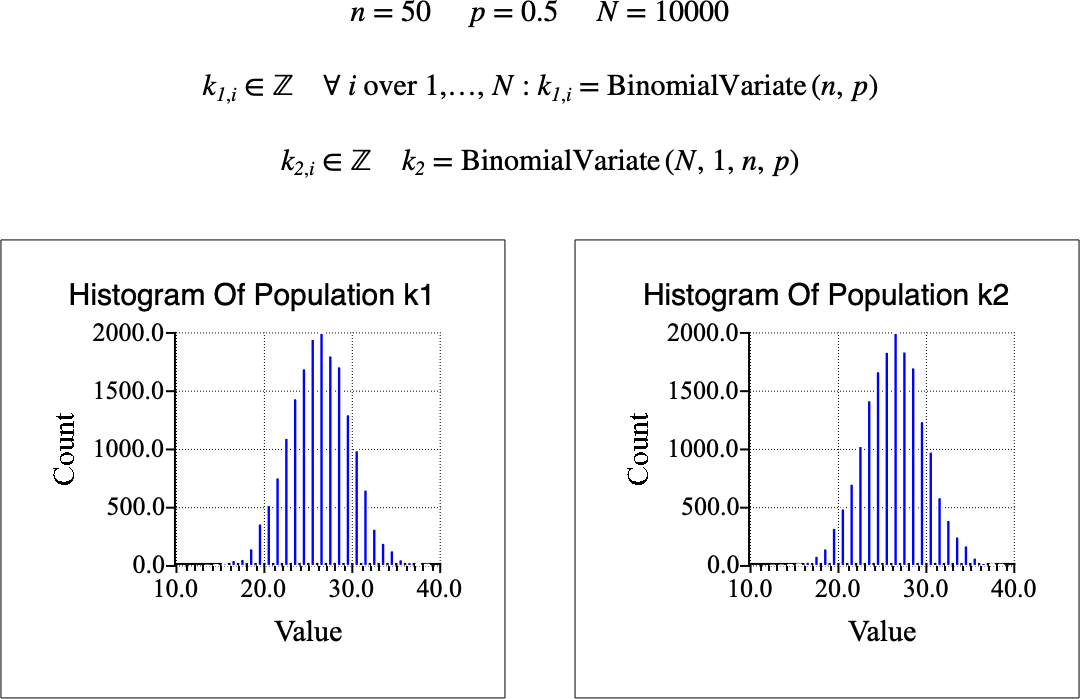# $$\text{BinomialVariate}$$¶

You can use the $$\text{BinomialVariate}$$ function to calculate one or more random variates in a binomial distribution.

You can use the \binomialv backslash command to insert this function.

The following variants of this function are available:

• $$\text{integer } \text{BinomialVariate} \left ( \text{<n>}, \text{<p>} \right )$$

• $$\text{integer matrix } \text{BinomialVariate} \left ( \text{<number rows>}, \text{<number columns>}, \text{<n>}, \text{<p>} \right )$$

Where $$n$$, and $$p$$ are scalar values. Note that this function is defined over the range $$k \geq 0$$, $$n \geq 0$$, and $$0 \leq p \leq 1$$ and will either generate a run-time error or return NaN, or a matrix of NaN for all other input values.

The four parameter version, which includes $$\text{<number rows>}$$ and $$\text{<number columns>}$$ fields, returns an integer matrix returning random deviates. The two parameter version returns a single value.

For small values of $$n$$ , less than 15, the $$\text{BinomialVariate}$$ uses an iterative approach based on the definition of the binomial distribution. While this may seem counter-intuitive, for small values of $$n$$, an iterative method is faster than the BTRD algorithm discussed below.

For values of $$n$$ equal to or greater than 15, the $$\text{BinomialVariate}$$ function employs the BTRD algorithm described in  which uses a transformed rejection method with decomposition (TRD).

The BTRD algorithm requires that a number of intermediate parameters be calculated from the inputs $$n$$, and $$p$$. To improve performance, our implementation of the BTRD algorithm uses memoization, storing all intermediate calculated parameters between calls, only updating those parameter values if the $$n$$ or $$p$$ values are different.

For this reason, if you need variates from multiple binomial distributions, you will obtain better performance if you use this function to calculate all the necessary values from one distribution before using this function to calculate values from a second or third distribution.

Figure 111 shows the two versions of the $$\text{BinomialVariate}$$ method in use.Figure 111 Example Use Of The BinomialVariate Function